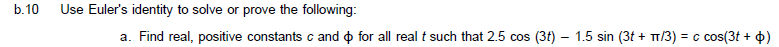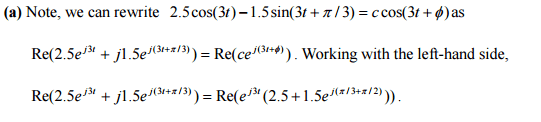# Signals and Systems Theory Question

OmniNewton

## Homework Statement[/B]How are we able to go from the first line to the second line and then the second line to the third?

## Homework Equations

Euler Identity: e^j(theta) = cos(theta) +jsin(theta)

## The Attempt at a Solution

This problem is more about preliminary theory in my opinion so I tried understanding how they converted the problem from trigonometric functions to exponential by analyzing the Euler Identity.

Last edited:

Homework Helper
Gold Member
Rewrite sin(x) and cos(x) in terms of the Euler identity, substitute in the original equation, and force equivalences.

•OmniNewton
OmniNewton
Rewrite sin(x) and cos(x) in terms of the Euler identity, substitute in the original equation, and force equivalences.

Thank you for the response sir but I really do not see how that works. How can one simply say that 2.5cos(3t) = 2.5e^(3jt). I thought cos(theta) = 1/2(e^j(theta) + e^-j(theta)) determined by the subtraction of 2 mcclauirin series.

Homework Helper
Gold Member
Thank you for the response sir but I really do not see how that works. How can one simply say that 2.5cos(3t) = 2.5e^(3jt).
You can't.
I thought cos(theta) = 1/2(e^j(theta) + e^-j(theta))
Right. Use that and the similar expression for sin(theta) and combine coefficients of ej3t and e-j3t.

•OmniNewton
OmniNewton
You can't. Right. Use that and the similar expression for sin(theta) and combine coefficients of ej3t and e-j3t.
Oh I see! That makes a lot of sense thank you kindly.